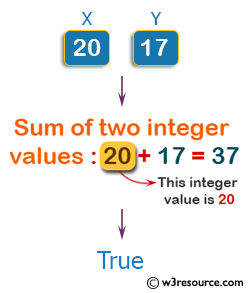﻿ Swift Basic Programming Exercise: Accept two integer values and return true if one of them is 20 or if their sum is 20 - w3resource# Swift Basic Programming Exercise: Accept two integer values and return true if one of them is 20 or if their sum is 20

## Swift Basic Programming: Exercise-3 with Solution

Write a Swift program that accept two integer values and return true if one of them is 20 or if their sum is 20.

Pictorial Presentation:Sample Solution:

Swift Code:

``````func make_20(x: Int, y: Int) -> Bool {
if x + y == 20 || x == 20 || y == 20
{
return true
}
else
{
return false
}
}

print(make_20(x: 29, y: 10))
print(make_20(x: 20, y: 17))
print(make_20(x: 11, y: 9))
```
```

Sample Output:

```false
true
true
```

Swift Programming Code Editor:

Improve this sample solution and post your code through Disqus

What is the difficulty level of this exercise?

﻿